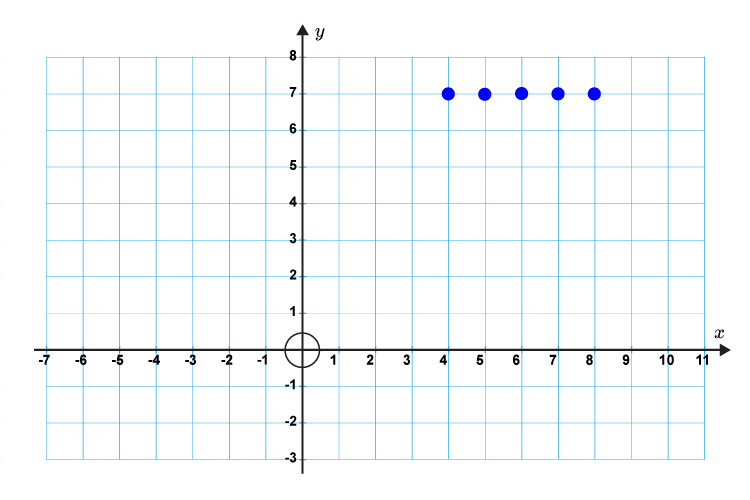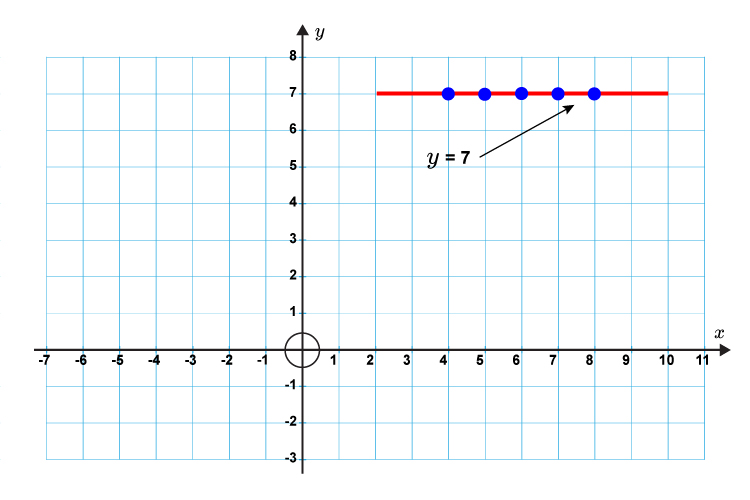# Equation of a horizontal line

The equation of a horizontal line is

y =  The same number

Example

y = 7

No matter what value x  is, y  is 7.

x = 2              y = 7

x = 1              y = 7

x = 0              y = 7

x = -1        y = 7

x = -2        y = 7

The graph would be plotted as follows:And would look like this.This is a horizontal line.